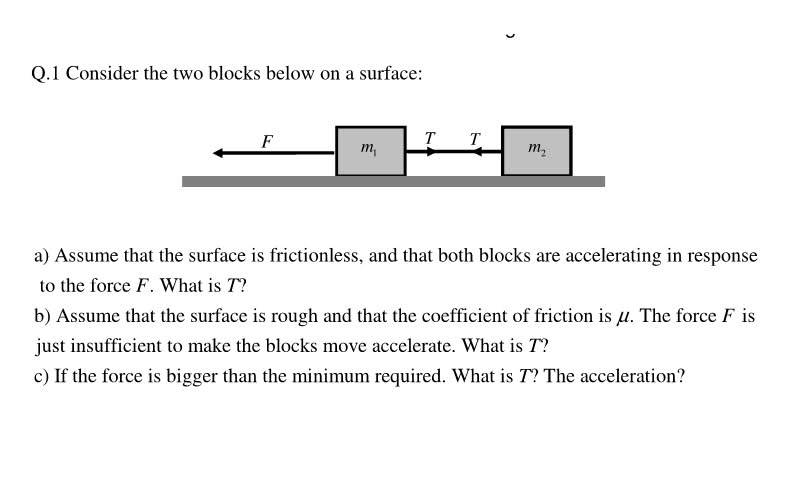# Two particles and a string.I need help with part c. Since there is now a rough surface does that mean the acceleration of the two particles will be different? Is it wrong to simply add up the two masses and pretend that its one mass. And then find the net friction force and use f=ma directly?
I don't understand in which cases I have to consider the two particles as one and in which cases separately?I need help with part c. Since there is now a rough surface does that mean the acceleration of the two particles will be different? Is it wrong to simply add up the two masses and pretend that its one mass. And then find the net friction force and use f=ma directly?
I don't understand in which cases I have to consider the two particles as one and in which cases separately?

No problem with considering them together when convenient.

Consider the combined mass and the combined friction in connection with the applied force, F, to find the acceleration.
Then use the Tension, the friction force on m2 the mass of m2 and the acceleration to find the value of T.

No problem with considering them together when convenient.

Consider the combined mass and the combined friction in connection with the applied force, F, to find the acceleration.
Then use the Tension, the friction force on m2 the mass of m2 and the acceleration to find the value of T.

I find the acceleration to be
a= [F-μ(m1+m2)g]/m1

Couldnt I also get another equation for a?

/e
Are you suggesting I use this a and put it in f=ma for m2?
Also since I can make FBD for either of the two masses and hence i could get two different equations for a. but both would be correct right?

I find the acceleration to be
a= [F-μ(m1+m2)g]/m1

Couldnt I also get another equation for a?

You are using a = F/m

I like your force [accounting for the friction on both masses]

I don't like your mass so much, as you have used only m1, rather than the combined mass.

You are using a = F/m

I like your force [accounting for the friction on both masses]

I don't like your mass so much, as you have used only m1, rather than the combined mass.

oh god. lol. sorry yes. the denominator should have the sum of the two masses. now how to approach the T
so this means there's only one correct equation for a. xd

oh god. lol. sorry yes. the denominator should have the sum of the two masses. now how to approach the T

having found a, now consider only mass m2 as T is the force that accelerates it.
This time you do only consider just one mass.

having found a, now consider only mass m2 as T is the force that accelerates it.
This time you do only consider just one mass.

Considering m2

T-Ff=m2a
T-μm2g=m2a

a= [F-μ(m1+m2)g]/(m1+m2)

Hence:
T= μm2g + m2[F-μ(m1+m2)g]/(m1+m2)

Hope I got it and typed it correct too. Thankyou for the help!